# Chain rule facts for kids

Kids Encyclopedia Facts

In differential calculus, the chain rule is a way of finding the derivative of a function. It is used where the function is within another function. This is called a composite function.

More specifically, if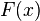$F(x)$ equals the composite function of the form: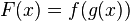$F(x)=f(g(x))$

where g is a function differentiable at x and f is a function differentiable at g(x), then the derivative of$F(x)$, written as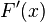$F'(x)$, exists, and is equal to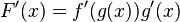$F'(x)=f'(g(x))g'(x)$.

## Steps

1. Find the derivative of the outside function (all of it at once).

2. Find the derivative of the inside function (the bit between the brackets).

3. Multiply the answer from the first step by the answer from the second step. This is basically the last step in solving for the derivative of a function.

For example,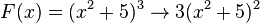$F(x) = (x^2+5)^3 \rightarrow 3(x^2+5)^2$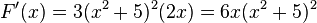$F'(x)=3(x^2+5)^2(2x) =6x(x^2+5)^2$

In this example, the cubed sign (3) is the outside function and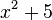$x^2+5$ is the inside function. The derivative of the outside function would be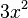$3x^2$, where x is replaced by the inside function. The derivative of the inside function would be 2x, which is multiplied by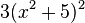$3(x^2+5)^2$ to get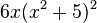$6x(x^2+5)^2$.

## Related pages

• Product ruleIn Spanish: Regla de la cadena para niños